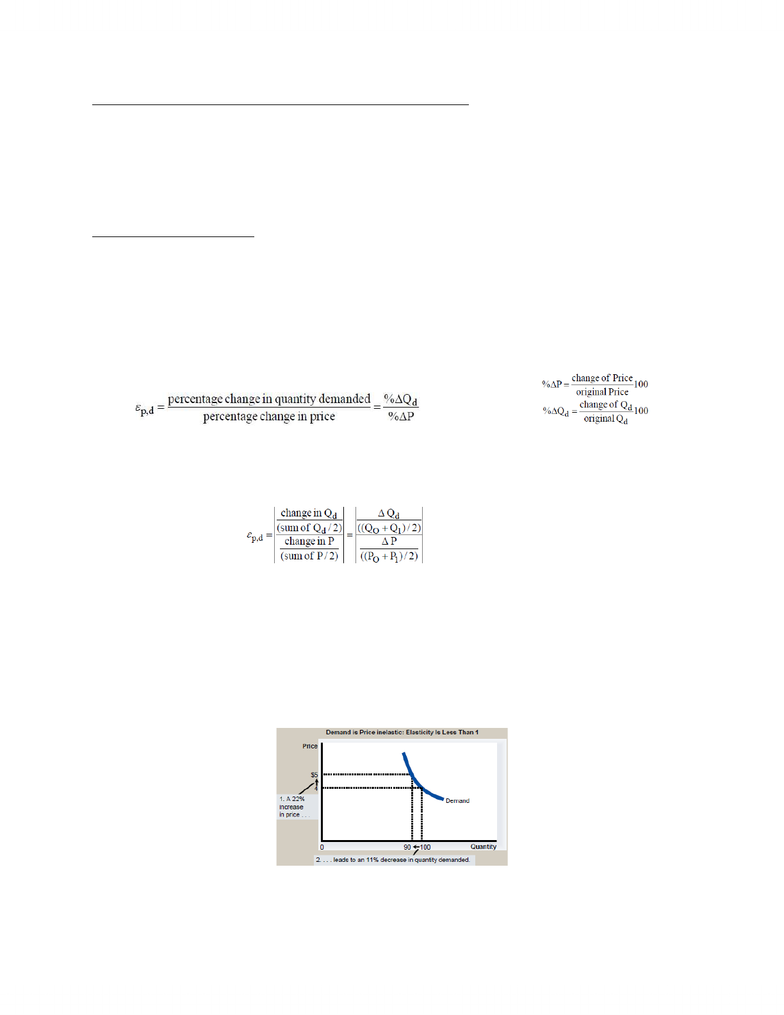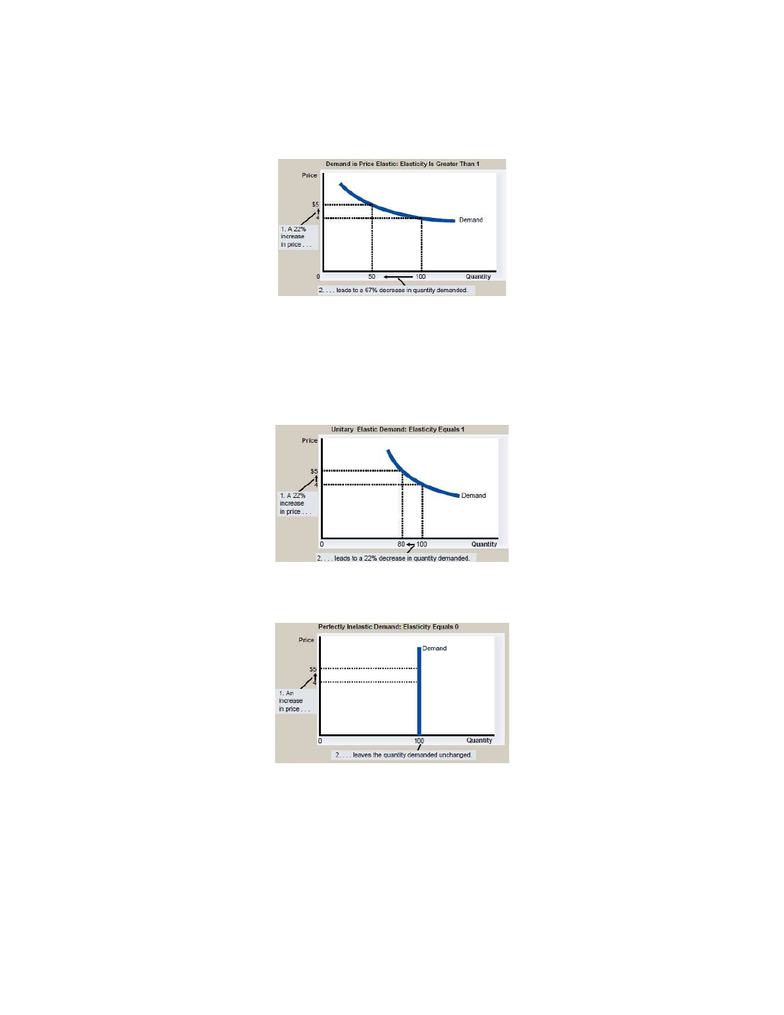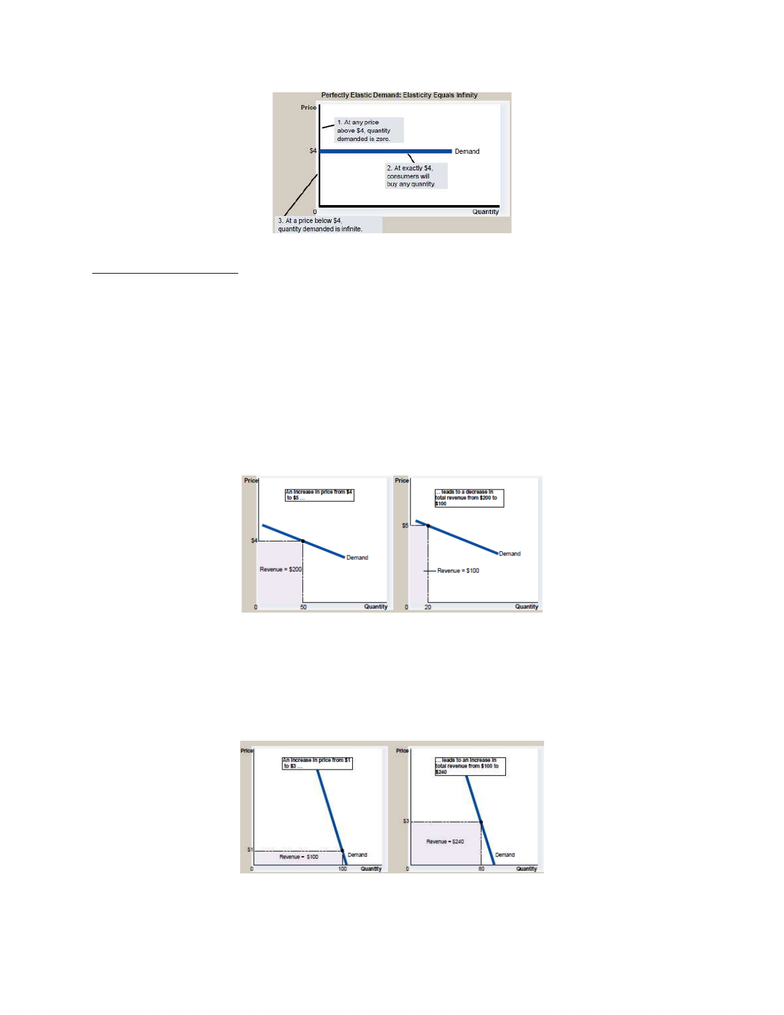Textbook Notes (280,000)
CA (160,000)
Ryerson (10,000)
ECN (800)
ECN 104 (300)
Chapter 4

# Chapter 4

Department
Economics
Course Code
ECN 104
Professor
Halis Yildiz
Chapter
4

This preview shows pages 1-3. to view the full 9 pages of the document.Chapter 4: Elasticity, Consumer Surplus and Producer Surplus
Elasticity allows analysis of supply and demand with greater precision
Measure of how many buyers & sellers respond to change in market
Elasticity: a measure of the responsiveness of QD/QS to one of its determinants
4.1 Price Elasticity of Demand
Price elasticity of demand: measure of the responsiveness of buyers to a change in the price of a
product/resource
Relatively elastic: QD responds STRONGLY to a change in price
Relatively inelastic: QD responds WEAKLY to a change in price
** take absolute value
Midpoint formula: method for calculating price elasticity of demand/supply that averages the two prices
& quantities a the reference points for competing %
**take absolute value
Interpretation of Price Elasticity of Demand
Elastic demand:
Quantity demanded responds strongly to changes in price
Product/resource demand with a price elasticity coefficient that is greater than one, Ed>1
Inelastic demand:
www.notesolution.com

Only pages 1-3 are available for preview. Some parts have been intentionally blurred.Quantity demanded doesnt respond strongly to price changes
Product/resource demand with a price elasticity coefficient that is less than one, Ed<1
Unit elasticity demand:
Quantity demanded changes by the same percentage as the price
price elasticity coefficient is equal to one, Ed = 1
Perfectly inelastic: quantity demanded doesnt respond to price changes
Perfectly elastic: quantity demanded changes infinitely with any change in price
www.notesolution.com

Only pages 1-3 are available for preview. Some parts have been intentionally blurred.4.2 The Total-Revenue Test
Total revenue: amount paid by buyers and received by sellers for a good TR = P x Q
Total revenue test: a test to determine elasticity of demand between any two prices, by noting what
happens total revenue when price changes
If TR changes in the opposite direction from price, demand is price elastic
increase in price decrease total revenue
% change in QD> % change in price
If TR changes in the same direction from price, demand is price inelastic
increase in price increase total revenue
%change in QD< % change in price
If TR doesn’t change when price changes, demand is unitary elastic
www.notesolution.com